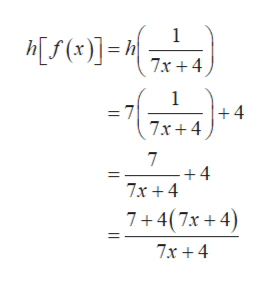# Givenh(x) = 7x + 4andf(x) =1/7x + 4,evaluate the composite function.h[f(x)]

Question
1 views

Given

h(x) = 7x + 4

and

f(x) =1/7x + 4,

evaluate the composite function.

h[f(x)]
check_circle

Step 1

Consider the function h(x)=7x+4 and f(x)=1/(7x+4).

Step 2

The composition of the function f(x) and g(x) is defined as,

Step 3

Now, the composition of the function h[f(...help_outlineImage Transcriptionclose1 (x)]- 7x 4 1 +4 7x+ 4 7 +4 7x 4 7+4(7x4 11 7x+ 4 fullscreen

### Want to see the full answer?

See Solution

#### Want to see this answer and more?

Solutions are written by subject experts who are available 24/7. Questions are typically answered within 1 hour.*

See Solution
*Response times may vary by subject and question.
Tagged in

### Algebra